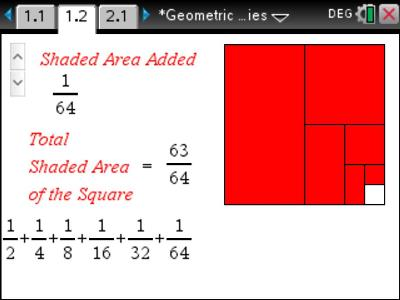•• ##### Device
•TI-Nspire™ CX
•TI-Nspire™ CX CAS
• ##### Software

TI-Nspire™
TI-Nspire™ CAS

4.5

# VIC: Geometric Sequences and Series

by Texas Instruments#### Objectives

• Generation of a geometric sequence and series using Sigma notation.
• Use the calculator to generate the formula for a Geometric Series
• Gain an understanding of Geometric Sequences and Series through powerful visuals.
• Use accurate mathematical notation.

#### Vocabulary

• Geometric Sequence
• Geometric Series
• Summation (Sigma Notation)
• Term
• Ratio